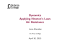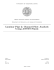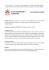# Homework#1 - Department of Mechanical Engineering

## Transcription

Homework#1 - Department of Mechanical Engineering
``` Eastern Mediterranean University
Faculty of Engineering
Department of Mechanical Engineering
Rigid Body Dynamics (MENG233)
Instructor:
Assistant Professor Dr. Mostafa Ranjbar
Assistant:
Homework #1
15th April 2015
Q1‐ A train starts from rest at station A and accelerates at 0.5 m/s2 for 60 s. Afterwards it travels
with a constant velocity for 15 min. It then decelerates at 1 m/s2 until it is brought to rest at
station B. Determine the distance between the stations.
Q2- The position of a particle on a straight line is given by S=(t3-9t2+15t) ft where t is in
seconds. Determine the position of the particle when t=6s and the total distance it travels during
the 6-s time interval. Hint: Plot the path to determine the total distance traveled.
Q3- A golf ball is struck with a velocity of 80 ft/s as shown.
Determine the distance d to where it will land.
Q4- When the motorcyclist is at A, he increases
his speed along the vertical circular path at the
rate of (‫ݒ‬ሶ = (0.3t) ft/s2) where t is in seconds.
If he starts from rest at A, determine the magnitudes
of his velocity and acceleration when he reaches B.
Q5- A toboggan is traveling down along a curve which
can be approximated by the parabola y  0.01x 2 . Determine
the magnitude of its acceleration when it reaches point A,
where its speed is v A  10m / s and it is increasing at the
rate of vA  3m / s 2 .
Q6- Determine the displacement of the block B if A is pulled down 4 ft.
Q7- At what rate, and in which direction must weight
A move if weight B is to fall at a rate of 0.3 m/s?
Q8- Cars A and B are traveling around the circular race track.
At the instant shown, A has a speed of 90 ft/s and is
increasing its speed at the rate of 15 ft/s2 whereas B has a
speed of 105 ft/s and is decreasing its speed at 25 ft/s2.
Determine the relative velocity and relative acceleration of
car A with respect to car B at this instant.
Q9- At the instant shown, cars A and B travel at speeds of
70 mi/h and 50 mi/h, respectively. If B is increasing its
speed by 1100 mi/h2, while A maintains a constant speed,
determine the velocity and acceleration of B with respect to
A. Car B moves along a curve having a radius of curvature of
0.7 mi.
```

### Solving Literal Equations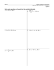### MAE 2600 (FS2014) Homework #18 Name: H18-1.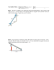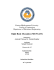### 2.1a Uniform Motion Grahps.notebook October 12, 2014 v =   d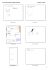### Throw a ball up and it will fall down 1) The force of gravity pulls the### Dynamic Second Exam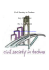### Chapter 5 Review Physics 11 1.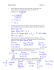### Speed, Velocity, and Acceleration Worksheet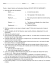### SAMPLE EXAM I: PHY 1114 Fall 2012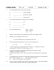### Linear Motion Test Review NOTES LOOK IT UP IN YOUR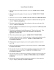### Rotational Kinematics Worksheet 1 Conversions: 1) Given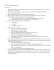### MAE420 Applied Fluid Mechanics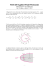### Past Final Exam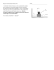### P1.2 Motion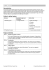### Remember EVERY ANSWER needs a number unit and direction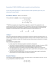### Sample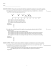### WHAT IS ACCELERATION?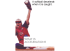### as a PDF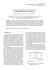### Rapid communication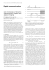### Lecture 17 - De Anza College